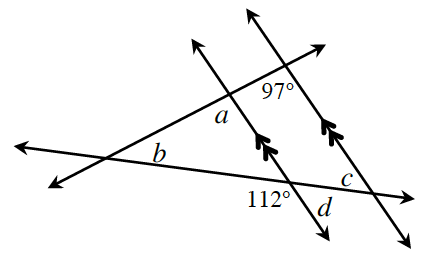### Home > CCG > Chapter 6 > Lesson 6.1.2 > Problem6-14

6-14.Examine the relationships that exist in the diagram at right. Find the measures of angles $a$, $b$, $c$, and $d$. Remember that you can find the angles in any order, depending on the angle relationships you use.

Notice the pair of parallel lines and the triangle.

Refer to the Math Notes box in Lesson 2.1.4 for extra help.